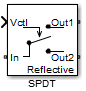# SPDT

Single pole double throw switch

## Library

Junctions

•## Description

The SPDT block models a single pole double throw switch. The input Simulink® signal, Vctl, controls the transfer of the signal at the input port, In, to one of the two output ports.

If the control voltage is less than the threshold voltage, the block transfers the input signal to output port 1. If control voltage is greater than or equal to the threshold voltage, the block transfers the input signal to output port 2. Two different resistors regulate the conduction path for transfer of input signal from input port to either output port.

The voltage–current relationship at the terminals depends on the relationship between control voltage, Vctl, and the threshold voltage, Vthreshold.

• If Vctl < Vthreshold:

`$\begin{array}{l}{I}_{in}={V}_{in1}.{G}_{on}^{}+{V}_{in2}.{G}_{off}\\ {I}_{1}\text{\hspace{0.17em}}\text{\hspace{0.17em}}\text{ }\text{ }=-{V}_{in1}.{G}_{on}\\ {I}_{2}\text{\hspace{0.17em}}\text{\hspace{0.17em}}=-{V}_{in2}.{G}_{off}+{V}_{2}.{G}_{Z02}\end{array}$`
• If VctlVthreshold:

`$\begin{array}{l}{I}_{in}={V}_{inout}.{G}_{off}^{}\\ {I}_{out}\text{\hspace{0.17em}}\text{\hspace{0.17em}}\text{ }\text{ }=-{V}_{inout}.{G}_{on}\end{array}$`

• Iin, I1 and I2 — Currents into the input terminal and two output terminals

• Vin1, Vin2 — Voltages between the input terminal and the output 1 and output 2 terminals, respectively

• V1, V2 — Voltages at output port 1 and output port 2, respectively

• Gon, Goff — On and off path conductances

• GZ01, GZ02 — Shunt port conductances

## Parameters

Threshold voltage

Threshold voltage of the switch, specified as a positive scalar. The default value is `0`  volts.

Characterization

Characterization of the `SPDT` switch: `Resistance` or ```Insertion loss```. The default value is `Resistance`.

On resistance

On resistance value of the switch, specified as a positive scalar. The default value is `10`  ohms. On resistance is available when you set Characterization to `Resistance`.

Off resistance

Off resistance value of the switch, specified as a positive scalar. The default value is `1e6`  ohms. Off resistance is available when you set Characterization to `Resistance`.

Insertion loss (dB)

Insertion loss value of the switch, specified as a positive scalar. The default value is `1`  dB. Insertion loss (dB) is available when you set Characterization to ```Insertion loss```.

Isolation (dB)

Isolation value of the switch, specified as a positive scalar. The default value is `70`  dB. Isolation (dB) is available when you set Characterization to `Insertion loss`

Loading type of the `SPDT` switch: `Absorptive` or `Reflective`. The default is `Reflective`.

Port terminations

Port termination that matches the impedance conditions of the `SPDT` switch. The default value is `50`  ohms.

### Note

Port terminations is available when you set the `SPDT` switch Characterization to `Insertion loss` or when you set Loading type to `Absorptive`.

Ground and hide reference terminals

Select this option to internally ground and hide the reference terminals. Clear the option to expose the reference terminals. By exposing these terminals, you can connect them to other parts of your model.

By default, this option is selected.